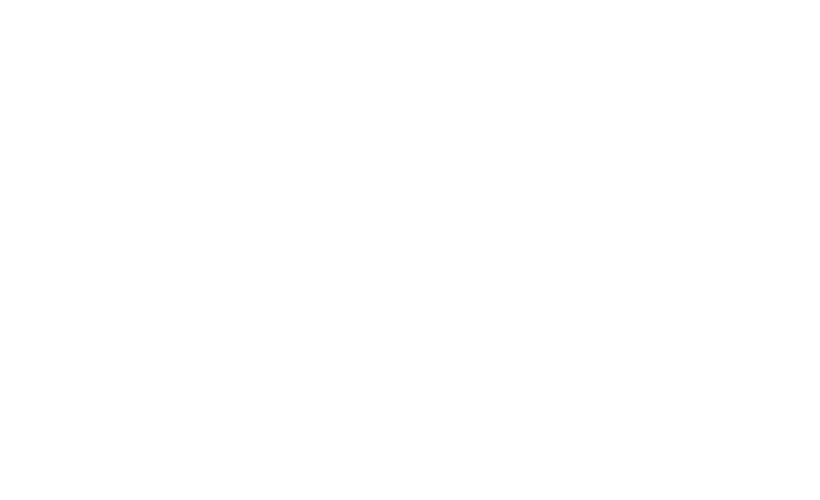# A simulation that shows how lenses diffract lightA simulation that shows how lenses diffract light

## 1 Comment

1.cenit997 says:

Clip extracted from a [video I made](https://www.youtube.com/watch?v=G4J4PV6tqH0), in which I explain the topic further with more simulations.

Diffraction is the physical phenomenon that limits the highest resolution obtainable with any optical system like in a microscope and this limit with a single lens can be estimated with the formula shown at the end of the clip:

d = λ *zi / r

where:

λ = wavelength of the light

zi = distance from the lens to the image

r = radius of the lens pupil

The lens pupil acts as a low pass filter, removing any spatial frequency of the image higher than r / (λ *zi )

While I used it with coherent light, it can be generalized to incoherent light by multiplying by 1/2, and it’s called **Abbe diffraction limit**.

To make the simulation reproducible, I also uploaded the source code [on GitHub](https://github.com/rafael-fuente/Diffraction-Simulations–Angular-Spectrum-Method/blob/main/Simulations%20with%20lenses.md). (optical_imaging_system.py)